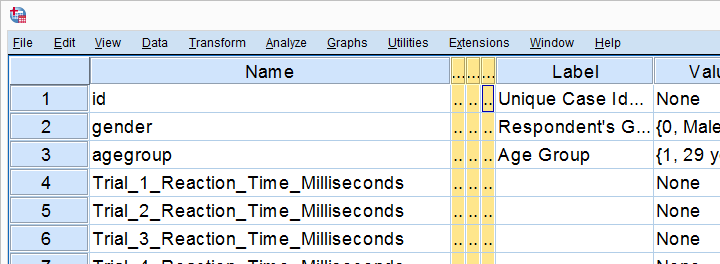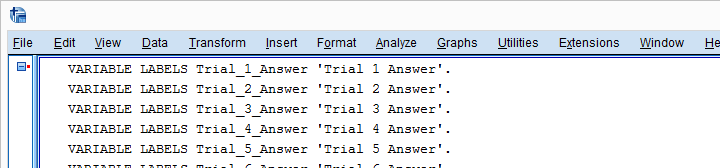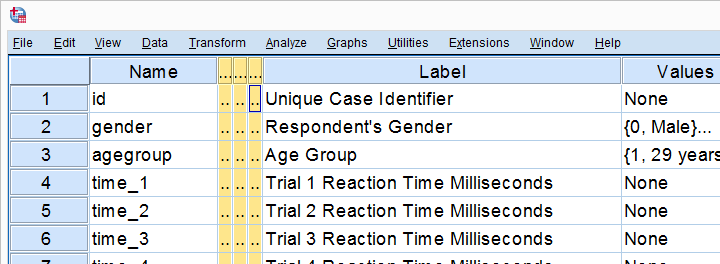SPSS TUTORIALS BASICS ANOVA REGRESSION FACTOR CORRELATION

# Set SPSS Variable Names as Labels with Python

Our previous lesson started out with a rather problematic data file. We reordered our variables with Python and saved our data as trials-ordered.sav, the starting point for this lesson. The screenshot below shows part of the data.We find the long variable names problematic for two reasons: first, typing them into a syntax window is too much work and results in overly long, unmanageable syntax. More importantly, the underscores don't look nice in our output but variable names can't hold spaces instead.
We'll therefore set our variable names as variable labels and replace the underscores by spaces. Finally, we'll replace the long names by nice and short ones.

## 1. Retrieve All Variable Names from Data

Retrieving all variable names from our data is a standard technique that we cover in Sort Variables in SPSS with Python. We'll do it with the syntax below once again.

*Look up all variable names.

begin program python3.
import spss
for ind in range(spss.GetVariableCount()):
varNam = spss.GetVariableName(ind)
print(varNam)
end program.

## 2. Retrieve All Variable Labels from Data

Note that our first 3 variables already have a label. In order to ensure we don't overwrite them, we'll now inspect all variable labels as well, which is a simple function covered by the spss module.

*Look up all variable labels.

begin program python3.
import spss
for ind in range(spss.GetVariableCount()):
varNam = spss.GetVariableName(ind)
varLab = spss.GetVariableLabel(ind)
print(varLab)
end program.

## 3. Create Variable Labels with Python

If some variable does not have a label yet, Python will return an empty string. We'll check if this holds with `if not varLab:`, which is `True` if the label is empty. For those variables, we'll create a variable label by replacing the underscores in their names by spaces. For now, we'll just print these labels.

*If variable label empty, variable label = variable name with underscores replaced by spaces.

begin program python3.
import spss
for ind in range(spss.GetVariableCount()):
varNam = spss.GetVariableName(ind)
varLab = spss.GetVariableLabel(ind)
if not varLab: # True if varLab is empty string (no VARIABLE LABEL set)
varLab = varNam.replace("_"," ")
print(varLab)
end program.

## 4. Create VARIABLE LABELS Commands

We'll now create and inspect VARIABLE LABELS commands with "VARIABLE LABELS %s '%s'."%(varNam,varLab) The first `%s` is replaced by the variable name, the second by its newly created label. This technique is explained in SPSS Python Text Replacement Tutorial.

*Create and inspect required VARIABLE LABELS commands.

begin program python3.
import spss
for ind in range(spss.GetVariableCount()):
varNam = spss.GetVariableName(ind)
varLab = spss.GetVariableLabel(ind)
if not varLab:
varLab = varNam.replace("_"," ")
print("VARIABLE LABELS %s '%s'."%(varNam,varLab))
end program.

Note: since we use single quotes around the variable label, there may be no single quotes within it. This is no issue here but if it is, escape each single quote within the label with 2 single quotes.

## Result## 5. Run VARIABLE LABELS Commands

Since our VARIABLE LABELS commands look fine, we'll now have Python run them in SPSS. We basically just replace `print` with `spss.Submit` and add some parentheses. This concludes the first part of our job.

*Have Python run VARIABLE LABELS commands.

begin program python3.
import spss
for ind in range(spss.GetVariableCount()):
varNam = spss.GetVariableName(ind)
varLab = spss.GetVariableLabel(ind)
if not varLab:
varLab = varNam.replace("_"," ")
spss.Submit("VARIABLE LABELS %s '%s'."%(varNam,varLab))
end program.

## 6. RENAME VARIABLES

At this point, at least our output will look good if we display only variable labels (not names) with SET TVARS LABELS. However, we still prefer nice and short variable names. If we use the TO keyword, a single line RENAME VARIABLES command does the trick for us.

*Set nice, short variable names for reaction times and answers.